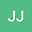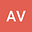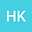The relativistic feature of Hydrogen-like atoms in Heisenberg picture
•••A Vahedi
Kharazmi University
Author ProfileH . Khosrojerdi
Kharazmi University
Author Profile## Abstract

The relativistic behavior of Hydrogen-like atoms (HLAs) is investigated in Heisenberg picture for the first time. The relativistic vibrational Hamiltonian (RVH) is first defined as a power series of harmonic oscillator Hamiltonian by using the relativistic energy eigenvalue . By applying the first-order RVH (proportional to ) to Heisenberg equation, a pair of coupled equations is turned out for the motion of electron position and its relativistic linear momentum. A simple comparison of the first-order relativistic and nonrelativistic equations reveals this reality that the natural (fundamental) frequency of electron oscillation (like entropy) is slowly raised by increasing the atomic number. The second-order RVH (proportional to ) have then been implemented to determine an exact expression for the electron relativistic frequency in the different atomic energy levels. In general, the physical role of RVH is fundamental because it not only specifies the temporal relativistic variations of position, velocity, and linear momentum of oscillating electron, but also identifies the corresponding relativistic potential, kinetic, and mechanical energies. The results will finally be testified by demonstrating the energy conservation.